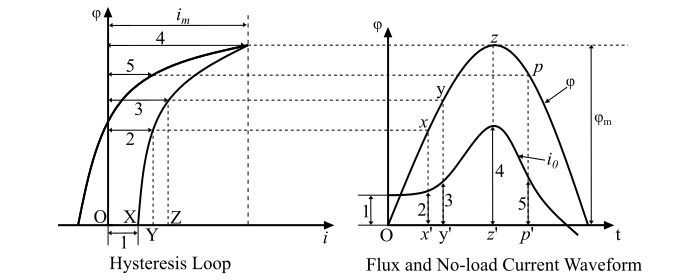# Transformer No-Load Current Wave Shape Considering Core Loss

A transformer needs less magnetic material if it is operated at a higher core flux density. Thus, from the economic point of view, a transformer is designed to operate in the saturation region of the magnetic core.

When a sinusoidal voltage is applied to the primary winding of the transformer, then the mutual flux set-up in the core is assumed to be sinusoidal and the no-load or exciting current (I0) will be non-sinusoidal due to hysteresis loop. It contains fundament and all odd harmonics.

Consider the hysteresis loop of the core of the given transformer as shown in the figure below. Since, the flux and current is given by

$$\mathrm{φ = 𝐵𝐴\:\:and\:\:𝑖 =\frac{𝐻𝑙}{𝑁}}$$

Therefore, the hysteresis loop is plotted in terms of flux (φ) and current (i) instead of B and H so that the current required to produce a particular value of magnetic flux can be determined directly. The waveform of the no-load current (I0) can be determined from the waveform of the magnetic flux and the φ-i curve of the magnetic core.In the φ-t curve, the flux at point O is zero. This value corresponds to a current OX on the hysteresis loop. The flux φ = xx’, at point ‘x’ of the φ-t curve and this corresponds to a current OB on the hysteresis loop. Likewise, the various abscissas of the φ-i curve are plotted as ordinates to determine the shape of the current wave on the φ-t curve. This procedure is followed round the whole loop until a sufficient number of points being obtained.

The waveform of the no-load current (i0) is shown in the figure above. Here, the waveform represents the magnetising component and the hysteresis component of the no-load current. The exciting current attains its maximum value at the same time as the magnetic flux wave, but the two waves do not go through zero simultaneously.

It can also be seen that the waveform of the no-load current of the transformer contains 3rd, 5th and higher-order odd harmonics which increase rapidly if the maximum flux is taken further into saturation, although the third harmonic is predominant one. For all the practical purposes, the harmonics higher than third harmonic are negligible. At rated voltage, the 3rd harmonic in the no-load current is about 5 to 10 % of the fundamental component.

Under load conditions, the total primary current is the phasor sum of no-load current and the load current. Since the load current is very large in magnitude as compared to the no-load current, the primary current is almost sinusoidal under load conditions of the transformer.